output.to from Sideway
Mechanics: Statics

Draft for Information Only

Content

Second Moment of Mass
Moment of Inertia of a Mass of Body
Radius of Gyraton of Mass of a Body
Moment of Inertia of Mass in Space
Parallel-Axis Thorem of Mass Moment of Inertia
Mass Moment of Inertia of a Three Dimensional Body of Homogenous Material

Second Moment of Mass

In general, the second moment of mass in space about an axis is also equal to the summation of the products of the square of the distance between the elemental mass and the reference axis, and the elemental mass over an area. As the second moment of mass is usually refered to the rotation, the distance between the elemental mass and the rotating axis is denoted by r. Imply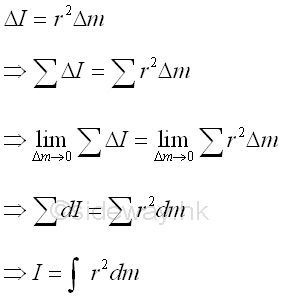Moment of Inertia of a Mass of Body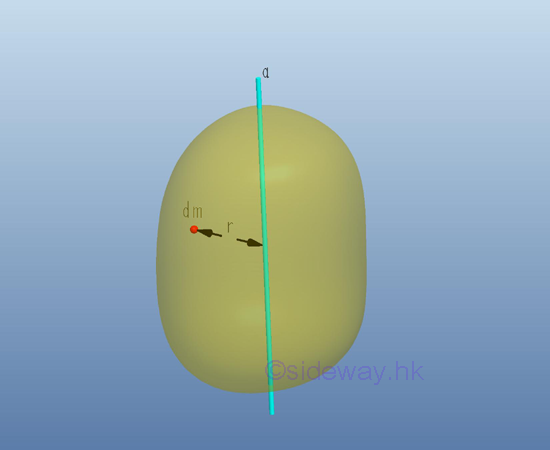Consider a body of mass m, the moment of inertia of mass of the solid body with respect to the axis a can also be determined by dividing the body into elemental mass Δm. The total moment of inertia of mass of the body, which is equal to the sum of all elemental mass, can then be obtained by taking limit as the number of elemental mass approach infinity. ImplyRadius of Gyraton of Mass of a Body

Similarly, the radius of gyraton k of the body is also defined as the distance between  the location of an imaginary total mass of the body and the reference axis such that an equivalent second moment of the mass is created by assuming the imaginary total mass of the body is placed at this distance away from the reference axis. Imply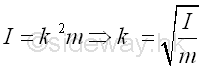Since the inertia of mass is related to the rotation of the body, the imaginary total mass m, used to represent the body, can be located at the perpendicular distance k anywhere away from the axis a for representing the same gyration or the rotation of the body about the axis a.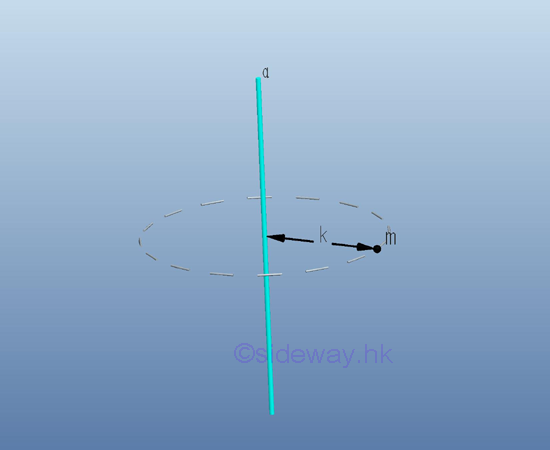Moment of Inertia of Mass in SpaceSimilar to the polar area moment of inertia in a rectangular coordinate system, the mass moment of inertia about an axis in space can also be expressed in terms of the elemental mass about an axis of the rectangular coordinate axes x, y, and z. as in the rectangular area moments of inertia. ImplyParallel-Axis Thorem of Mass Moment of Inertia

Consider the same body of mass m with centre of gravity G. Let the rectangular coordinate axes with origin O is XYZ and the parallel centroidal rectangular coordinate axes with origin G, at distance (x,y,z) away from origin O,  is X'Y'Z'. ImplyTherefore, the distance x,y,z between the elemental mass and the coordinate axes with origin O can be expressed in terms of the distance x', y',z, between the elemental mass and the coordinate axes with origin G. Imply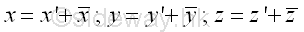Similarly the mass moments of inertia of the body about the rectangular coordinate axes with origin O can be expressed in terms of the mass moments of inertia of the body about the parallel centroidal rectangular coordinate axes with origin G also. ImplyThe integral of the moment of inertia I of the mass m about the rectangular axis  can be divided into four seperated integrals. The first integral is the centroidal moment of inertia I of the mass. The second and third integrals are the first moment of the mass m about the centroidal axis and the value of the first moment of the mass about the centroidal axis is equal to zero. The fourth integral is equal to the total mass only. Imply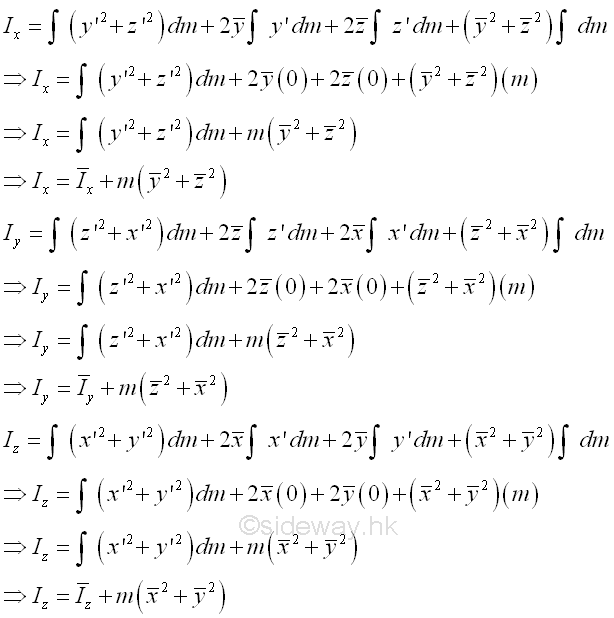The sum of the square of the rectangular coordinate distance from axes is equal to the square of the distance of the perpendicular displacement from the axes. ImplyOr the mass moment of inertia can be expressed in terms of the radii of gyration. Imply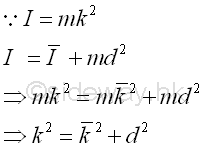Mass Moment of Inertia of a Three Dimensional Body of Homogenous Material

For a three-dimensional body made of homomgenous material of density ρ, the elemental mass dm can be expressed in terms of the volume of the three dimensional body. In other words, the mass moment of inertia of a three dimensional body of homogenous material density depends on the shape of the three dimensional body only. Imply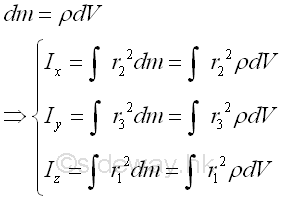References

1. I.C. Jong; B.G. rogers, 1991, Engineering Mechanics: Statics and Dynamics, Saunders College Publishing, United States of America
2. F.P. Beer; E.R. Johnston,Jr.; E.R. Eisenberg, 2004, Vector Mechanics for Engineers: Statics, McGraw-Hill Companies, Inc., New YorkID: 121100085 Last Updated: 2012/11/14 Revision: 0 Ref:Home (5)

Management

HBR (3)

Information

Recreation

Hobbies (7)

Culture

Chinese (1097)

English (336)

Reference (66)

Computer

Hardware (149)

Software

Application (187)

Digitization (24)

Numeric (19)

Programming

Web (704)CSS (SC)

ASP.NET (SC)

HTML

Knowledge Base

Common Color (SC)

Html 401 Special (SC)

OS (389)

MS Windows

Windows10 (SC)

.NET Framework (SC)

DeskTop (7)

Knowledge

Mathematics

Formulas (8)

Algebra (20)

Number Theory (206)

Trigonometry (18)

Geometry (18)

Calculus (67)

Complex Analysis (21)

Engineering

Tables (8)

Mechanical

Mechanics (1)

Rigid Bodies

Statics (92)

Dynamics (37)

Fluid (5)

Control

Acoustics (19)

Biology (1)

Geography (1)